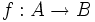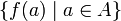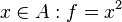Range

The range of a function$f:A \to B$ is the subset of$B$ given as$\{ f(a) \mid a \in A \}$, i.e., the set of elements of$B$ that arise as outputs of the function.
• Given$f:A \to B$ so that for every$x\in A: f=x^2$ in this case the range is a subset of$B$ encompassing all its positive real numbers including$0$.